• matlab 自适应图像增强算法 亲测有效 适合初学者作参考！
• 智能优化算法应用：基于麻雀搜索算法与双伽马校正的图像自适应增强算法 - 附代码 文章目录智能优化算法应用：基于麻雀搜索算法与双伽马校正的图像自适应增强算法 - 附代码1.全局双伽马校正2.麻雀搜索算法3.适应度...
智能优化算法应用：基于麻雀搜索算法与双伽马校正的图像自适应增强算法 - 附代码
文章目录智能优化算法应用：基于麻雀搜索算法与双伽马校正的图像自适应增强算法 - 附代码1.全局双伽马校正2.麻雀搜索算法3.适应度函数设计4.实验与算法结果5.参考文献6.Matlab代码
摘要：本文主要介绍基于麻雀搜索算法与双伽马校正的图像自适应增强算法。
1.全局双伽马校正
设图像的灰度值范围被归一化到[0, 1]范围之内，基于全局亮度的双伽马调整函数(Bilateral  Gamma  Adjustment,  BiGA)的图像增强方法该函数由 2个伽马函数Ga 和Gb 融合而成，其数学表达式如下：
$G_a(x) = x^{1/r} \tag{1}$
$G_b(x)=1-(1-x)^{1/r} \tag{2}$
$G(x) = \alpha G_a(x) + (1-\alpha)G_b(x) \tag{3}$
式中 $x$ 是输入图像的灰度值，$r$ 是可调节变量，用以调整图像增强程度，一般取 $r =2.5$，$\alpha$ 是调节参数，取值范围为[0, 1]。$G_a(x)$ 是一个凸函数，$G_b(x)$用于增强暗区域。 是一个凹函数，用于抑制图像的亮区域。最终 BiGA 增强算法的调整函数$G(x)$是由$G_a(x)$和$G_b(x)$ 取加权得到的。
使用 BiGA 对图像进行校正之前，首先利用式(4)进行归一化理，然后利用式(5)进行双伽马函数校正图像，最后，采用式(6)将其取值范围调整到[0,255]之间。
$I_1(x,y) = I(x,y)/256 \tag{4}$
$I_2(x,y)=G(I_1(x,y)) \tag{5}$
$I(x,y) = I_2(x,y)*256 \tag{6}$
2.麻雀搜索算法
麻雀搜索算法具体原理请参照：https://blog.csdn.net/u011835903/article/details/108830958。
3.适应度函数设计
本文评价函数设计如下：
$fitness = \alpha_1*H + \alpha_2*S + \alpha_3*log(Stv) \tag{7}$
其中$\alpha_1,\alpha_2,\alpha_3$为常数，代表目标函数的相对重要性。本文将熵值、边缘内容和灰度标准方差等同取值，即$\alpha_1 = \alpha_2 =\alpha_3 = 1/3$.
式(7)中 $H$ 代表测试图像的熵值，图像的熵值越大，代表图像所含的信息量越大，细节越丰富。
$H = -\sum_{i=0}^{255}p(i)log_2(p(i)) \tag{8}$
其中 $p(i)$某个灰度值($i$)在该图像中出现的概率。
式(7)中 $S$ 代表由 $sobel$ 边缘检测算子计算的测试图像的边缘内容，其值越大，代表测试图像包含的边缘信息越多，图像对比度越好。其定义式如下：
$S = \frac{n\_edges(E)}{T} \tag{9}$
$n\_edges(E)$表示由 sobel边缘检测算子检测到的增强图像中的边缘像素的数量，$T$ 是增强图像中的像素总数。
式(7)中 $Stv$ 为测试图像的灰度标准方差，一般情况下，$Stv$ 值越大，所测图像的对比度就越好，越适合人眼观察。
所以对于麻雀搜索算法，寻优即寻找使得$fitness$最大，转换成寻最小值，即$-fitness$最小。
4.实验与算法结果
麻雀搜索算法参数设定如下：
%设定麻雀算法参数
SearchAgents_no=30; %  种群数量
Max_iteration=50; %  设定最大迭代次数
lb = 0; %下边界
ub = 1;  %上边界
dim = 1; %1维度即alpha参数
fobj = @(X) fun(I,X);%适应度函数收敛曲线：5.参考文献
刘金华. 基于元启发式算法的低照度图像增强研究[D].郑州轻工业大学,2020.
 Sazzad  T  S,  Hasan  M  Z,  Mohammed  F,  et  al.  Gamma  encoding  on  image  processing considering  human  visualization,  analysis  and  comparison[J].  International  Journal  on Computer Science and Engineering, 2012, 4(12): 1868.
6.Matlab代码
https://mianbaoduo.com/o/bread/aZmZmJY=


展开全文算法 计算机视觉 人工智能 机器学习
• 图像的区域自适应增强的C++实现，效果跟matlab的区域自适应增强的函数一样
• 自适应图像增强的全称叫自适应直方图均衡，对应的是Matlab 中的adapthisteq 函数。 ​ 这种方法用直方图匹配方法来逐个处理图像中的较小区域（称为小块）。然后使用双线性内插方法将相邻的小片组合起来，从而...
自适应图像增强
​		自适应图像增强的全称叫自适应直方图均衡，对应的是Matlab 中的adapthisteq 函数。
​		这种方法用直方图匹配方法来逐个处理图像中的较小区域（称为小块）。然后使用双线性内插方法将相邻的小片组合起来，从而消除人口引入的边界。特别在均匀的灰度区域，可以限制对比度来避免放大噪声。
J = adapthisteq(I)
J = adapthisteq(I,param1,val1,param2,val2...)

灰度图像处理
I = imread('E:\txcl\image processing\NCST1.jpeg');
B=rgb2gray(I);
A = adapthisteq(B,'clipLimit',0.02,'Distribution','rayleigh');
C = adapthisteq(B);
subplot(2,2,1),imshow(I);
subplot(2,2,2),imshow(B);
subplot(2,2,3),imshow(A);
subplot(2,2,4),imshow(C);


注意：必须转化为二维图像才可以处理  其他图像不行

RGB图像处理（3维）
B = imread('E:\txcl\image processing\NCST1.jpeg');%后面除第三行改别的可以直接复制

subplot(221),imshow(B);

[X , MAP] = rgb2ind(B,255,'dither');
RGB = ind2rgb(X,MAP);
cform2lab = makecform('srgb2lab');
LAB = applycform(RGB, cform2lab);
L = LAB(:,:,1)/100;
LAB(:,:,1) = adapthisteq(L,'NumTiles',[8 8],'ClipLimit',0.005)*100;
cform2srgb = makecform('lab2srgb');
J = applycform(LAB, cform2srgb);

subplot(222),imshow(RGB);

subplot(223),imshow(J);


RGB图像转换为索引图像

直接调用rgb2ind()

参数dither表示是否使用消抖

[X,map]=rgb2ind(I,tol)

其中I是原RGB图像
tol的范围从0.0-1.0
[X,map]为生成的索引图像
map为索引图像的颜色表
X应该并且必须是二维图像

[X，map]=rgb2ind(I,N)

map中至少包含N个颜色

X=rgb2ind(I,map)

该函数是通过与RGB中最相近的颜色进行匹配生成颜色映射表map
[X,map]对应于新的索引图像

​


展开全文• 对高通子带则根据人类视觉特性定义局部方向对比度,并作为 融合准则,突出和增强了各源图像的对比度与细节信息.实验结果表明:与基于小波的融合结果相比较,本文的融合算法自适应性和鲁棒性更强,较好地保护和显示 了源...
• 自适应直方图均衡化、同态滤波、自适应对比度增强matlab源代码，已经本人测试
• matlab开发-用于增强彩色图像的强度和基于差分的自适应非锐化滤波器。基于强度和边缘的彩色图像增强自适应不清晰度掩蔽滤波器
• matlab开发-用于增强图像对比度的不清晰度屏蔽滤波器的自适应比例调整设计。图像对比度增强非锐化掩模滤波器的自适应尺度调整设计
• 仿真结果 在实际视频的处理过程中，参数的调整如何做到自适应，则是一个必须考虑的问题。...是不是要针对所有的边缘进行增强图像存在强边缘和弱边缘的。 边缘增强的幅度，考虑undershot 和 ove...
仿真结果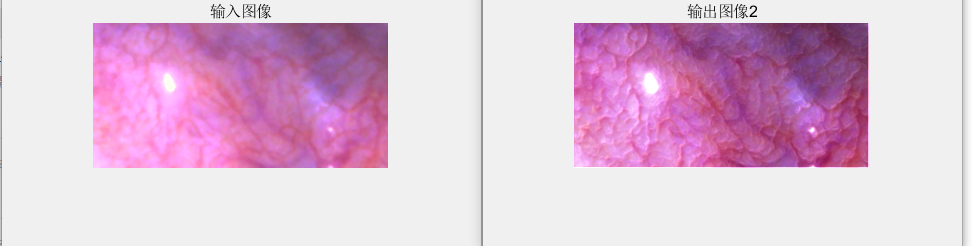在实际视频的处理过程中，参数的调整如何做到自适应，则是一个必须考虑的问题。Video Processing和Image Processing不完全相同。

边缘增强的第一步是找到需要增强的高频分量，但是要明白，不是所有高频分量都是边缘，有一类噪声也属于高频分量。

是不是要针对所有的边缘进行增强，图像存在强边缘和弱边缘的。

边缘增强的幅度，考虑undershot 和 overshot

基础的图像处理调试必然是基于大量重复工作得到一组最优的参数，时刻保持好奇心，去寻找心中那组最优的参数。


展开全文• 今天写调用摄像头处理图像函数，用到了adapthisteq函数，顺便...与histeq函数不同的是，adapthisteq处理的是图片的小块区域的数据，而不是整个图像对比度增强，使输出区域的直方图大约匹配指定的直方图。相邻的小块...
今天写调用摄像头处理图像函数，用到了adapthisteq函数，顺便对adapthisteq函数做了一些总结。
adapthisteq：适应直方图均衡化对比度限制器。通过转换强度图像中的值来改变图像的对比度。
histeq：自适应直方图均衡。
与histeq函数不同的是，adapthisteq处理的是图片的小块区域的数据，而不是整个图像对比度增强，使输出区域的直方图大约匹配指定的直方图。相邻的小块用双线性插值把它们混合在一起，以消除人工诱导的边界。对比，尤其是在同质区域，可以限制，以避免放大图像中可能存在的噪声。
G = adapthisteq(I, param1, val1, param2, val2, …)
’NumTiles’：由正整数组成的二元向量[ m, n ]。[ m, n ]为指定小块区域的行列数，M 和 n 都必须至少为2。图像块的总数等于 m * n。 默认值为 [ 8,  8 ]。
’ClipLimit’  ：是从0到1的实数标量，用于限制对比度增强。数值越大，对比度越高。默认值为: 0.01。
’NBins’：正整数标量。为构建直方图时使用的直方图设置容器数增强对比度的转换。更高的值结果导致有在更大的动态范围，代价是较慢的处理速度。 默认值: 256。
’Range’：‘original’ 或 ‘full’. 控制输出图像数据的范围。 如果‘ Range’设置为‘ original’ ，则范围限制为[ min (i (：))  max (i (：))]。 否则，默认情况下，或者当‘ Range’设置为‘ full’时，将使用输出图像类的全部范围(e.g.    uint8  是 [0 255])。默认值：full。
’Distribution’：‘uniform’, ‘rayleigh’, ‘exponential’。通过指定分布类型，为图像块设置所需的直方图形状。 默认值：uniform。
’Alpha’：是一个分布参数，当“ Dist”为“ rayleigh”或“ exponential”时可以使用这个参数。 默认值：0.4。
下面是随手写的一份代码，可以直观的看到adapthisteq的使用方法和使用效果。
I = rgb2gray(imread('tupian.png'));
figure;
imshow(I);

I1 = adapthisteq(I);
figure;
imshow(I1);

I2 = adapthisteq(I,'NumTiles',[50 50]);
figure;
imshow(I2);

I3 = adapthisteq(I,'NumTiles',[50 50],'ClipLimit',0.5);
figure;
imshow(I3);

I4 = adapthisteq(I,'NumTiles',[50 50],'ClipLimit',...
0.5,'NBins',400);
figure;
imshow(I4);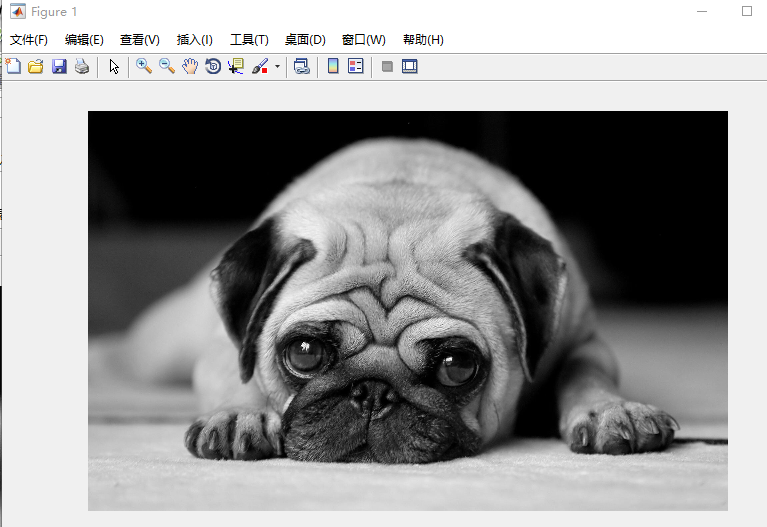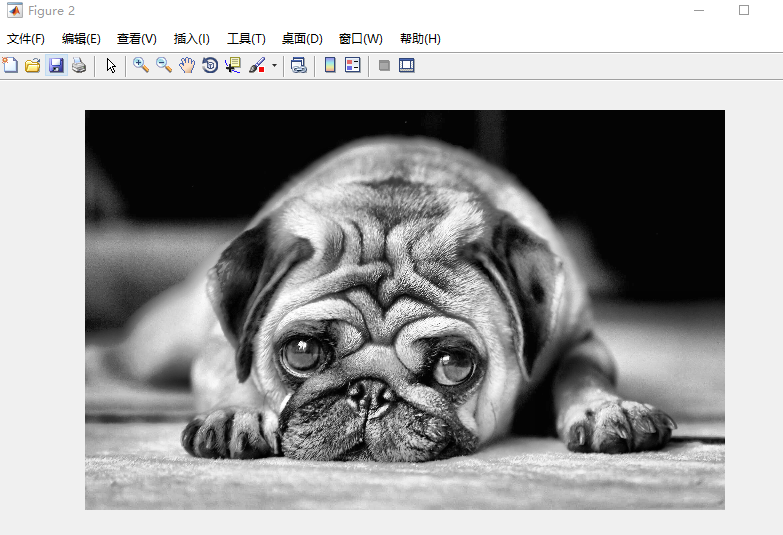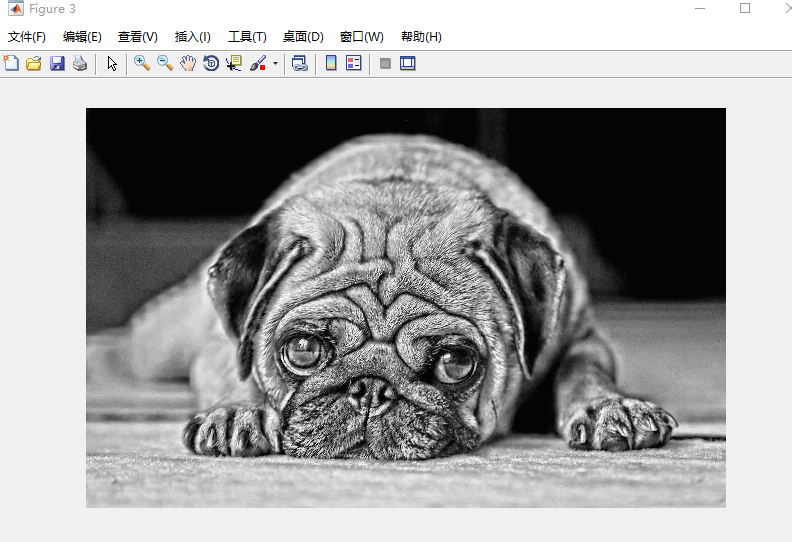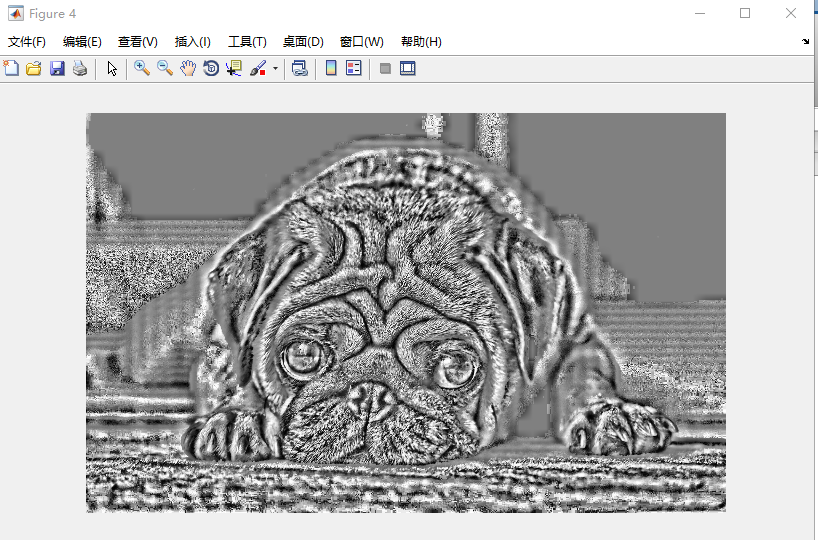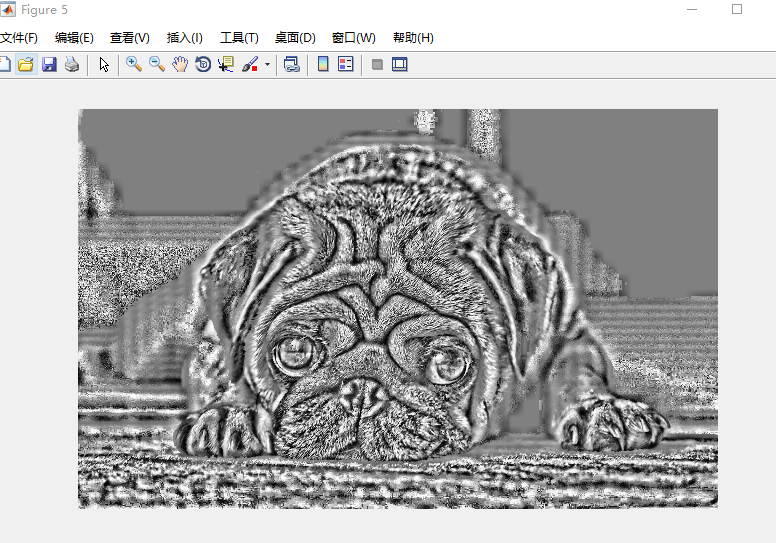这里注意第一行代码，
I = rgb2gray(imread('tupian.png'));
I = imread('tupian.png');

很多人会忘记做灰度处理导致adapthisteq使用错误。
写文章时间较紧，如有错误，还请指正。


展开全文• 智能优化算法应用：基于麻雀搜索...摘要：本文主要介绍如何用麻雀搜索算法和非完全beta函数实现图像自适应增强。 1.图像非线性增强 图像像素灰度变换可表示为： Ixy∗=f(ixy)(1) I_{xy}^* = f(i_{xy}) \tag{1} Ixy∗算法 计算机视觉 人工智能 机器学习
• 对于整体亮度较弱的图像，我们常常需要进行增强，以下是采用自适应方式进行图像处理。 close all; clear all; warning off all; I = imread('rice.png'); figure; imshow(I); title('原始图像'); [m,n,d] = size...基础教程
• 1、decorrstretch 使用去相关拉伸图像增强 不太明白嘛意思 2、adapthisteq  作用 有限对比度自适应直方图均衡化 3、hsiteq 直方图均衡化 作用 使用直方图均衡化加强图像的对比度 J=histeq(I...
• 一、简介 基于模糊集的图像增强主要包括三个步骤：图像模糊特征提取、隶属...现有的方法自适应的效果都比较差，这里提出一种模糊自适应的方法，利用遗传算法完成图像增强。 1，选着合适的评价函数，得到图像质量的适
• (1)数字图像矩阵傅立叶变换(2)图像压缩(3)增强图像对比度(4)直方图均匀化(5)图像样本增强(6)图像滤波(7)图像均值滤波(8)图像自适应魏纳滤波(9)5种梯度增强法图像锐化(10)图像的高通滤波和掩模处理(11)图像平滑处理...
• matlab开发-灰度图像增强剂。基于自适应乙状结肠函数的双柱状图均衡图像增强
• P0307：采用MATLAB中的函数filter2对受噪声干扰的图像进行均值滤波 P0308：图像自适应魏纳滤波 P0309：运用5种不同的梯度增强法进行图像锐化 P0310：图像的高通滤波和掩模处理 P0311：利用巴特沃斯...
• 最大类间方差（OSTU 自适应阈值提取 图像边缘增强算法 MATLAB代码ffff
• 分别用直方图，自适应，对比度调整方法实现彩色图像增强，有代码，有图片
• 分别用直方图，自适应，对比度调整方法实现彩色图像增强，有代码，有图片 分别用直方图，自适应，对比度调整方法实现彩色图像增强，有代码，有图片
• 本程序实现了图像处理的几个功能，如图像增强图像安全、图像复原。其中用的算法有灰度变换增强、直方图均衡化处理、中值滤波滤波、均值滤波、顺序滤波、自适应滤波等
• matlab图像分割什么是图像分割固定阈值分割自适应阈值分割分割效果增强平滑处理 什么是图像分割 将数字图像划分成不相交，不重合的区域的过程就叫图像分割。 这很像PhotoShop里面的快速选取工具，判断边界，分割边界...
• 一、简介 基于模糊集的图像增强主要包括三个步骤：图像模糊特征提取、隶属函...现有的方法自适应的效果都比较差，这里提出一种模糊自适应的方法，利用遗传算法完成图像增强。 1，选着合适的评价函数，得到图像质量的
• matlab代码采用小波变换的图像增强自适应算法，效果很好。小波
• P0307：采用MATLAB中的函数filter2对受噪声干扰的图像进行均值滤波 P0308：图像自适应魏纳滤波 P0309：运用5种不同的梯度增强法进行图像锐化 P0310：图像的高通滤波和掩模处理 P0311：利用巴特沃斯...
• ACE前言原理代码实验结果 前言 现如今的医学图像 原理 代码 实验结果计算机视觉
• 一、简介 基于模糊集的图像增强主要包括三个步骤：图像模糊特征提取、隶属...现有的方法自适应的效果都比较差，这里提出一种模糊自适应的方法，利用遗传算法完成图像增强。 1，选着合适的评价函数，得到图像质量的适
• 同时针对设计出来的奇异值分解的图像压缩算法进行性能分析与研究，发现可以通过自适应中值滤波进行图像优化，增强图像的视觉清晰度；还可以在对图像进行小波变换，从而进行图像频域提取，达到小波分解的效果。利用小...# matlab图像自适应增强matlab 订阅